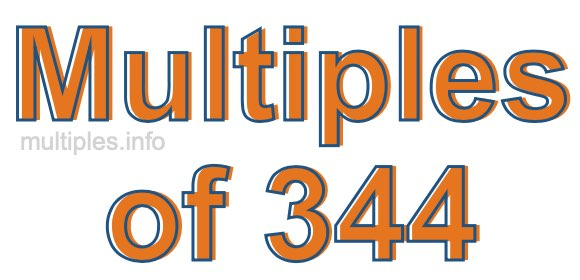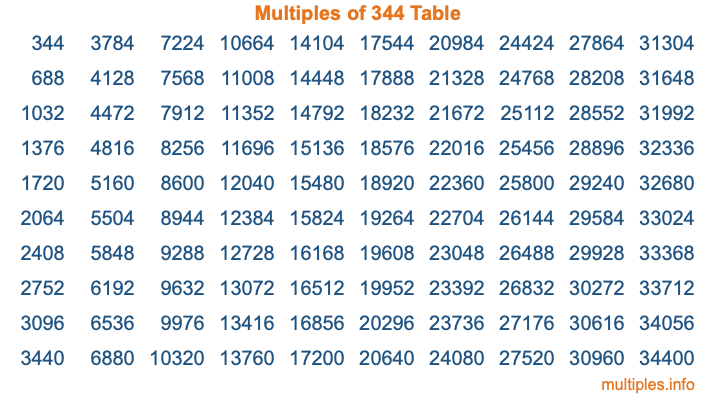Multiples of 344Welcome to the Multiples of 344 page. Here we will first teach you everything you will ever need to know about the multiples of 344, and then give you a study guide summary of everything we taught you to make sure you remember it all. Use this page to look up facts and learn information about the multiples of 344. This page will make you a multiples of three hundred forty-four expert!

Definition of Multiples of 344
Multiples of 344 are all the numbers that when divided by 344 equal an integer. Each of the multiples of 344 are called a multiple. A multiple of 344 is created by multiplying 344 by an integer.

Therefore, to create a list of multiples of 344, you start with 1 multiplied by 344, then 2 multiplied by 344, then 3 multiplied by 344, and so on for as long as you want. Thus, the list of the first five multiples of 344 is 344, 688, 1032, 1376, and 1720. To see a larger list of multiples of 344, see the printable image of Multiples of 344 further down on this page. We also have a category where you can choose any nth multiple of 344.

Multiples of 344 Checker
The Multiples of 344 Checker below checks to see if any number of your choice is a multiple of 344. In other words, it checks to see if there is any number (integer) that when multiplied by 344 will equal your number. To do that, we divide your number by 344. If the the quotient is an integer, then your number is a multiple of 344.

Is  a multiple of 344?

Least Common Multiple of 344 and ...
A Least Common Multiple (LCM) is the lowest multiple that two or more numbers have in common. This is also called the smallest common multiple or lowest common multiple and is useful to know when you are adding our subtracting fractions. Enter one or more numbers below (344 is already entered) to find the LCM.

Check out our LCM Calculator if you need more details about the Least Common Multiple or if you need the LCM for different numbers for adding and subtraction fractions.

nth Multiple of 344
As we stated above, 344 is the first multiple of 344, 688 is the second multiple of 344, 1032 is the third multiple of 344, and so on. Enter a number below to find the nth multiple of 344.

th multiple of 344

Multiples of 344 vs Factors of 344
344 is a multiple of 344 and a factor of 344, but that is where the similarities end. All postive multiples of 344 are 344 or greater than 344. All positive factors of 344 are 344 or less than 344.

Below is the beginning list of multiples of 344 and the factors of 344 so you can compare:

Multiples of 344: 344, 688, 1032, 1376, 1720, etc.

Factors of 344: 1, 2, 4, 8, 43, 86, 172, 344

As you can see, the multiples of 344 are all the numbers that you can divide by 344 to get a whole number. The factors of 344, on the other hand, are all the whole numbers that you can multiply by another whole number to get 344.

It's also interesting to note that if a number (x) is a factor of 344, then 344 will also be a multiple of that number (x).

Multiples of 344 vs Divisors of 344
The divisors of 344 are all the integers that 344 can be divided by evenly. Below is a list of the divisors of 344.

Divisors of 344: 1, 2, 4, 8, 43, 86, 172, 344

The interesting thing to note here is that if you take any multiple of 344 and divide it by a divisor of 344, you will see that the quotient is an integer.

Multiples of 344 Table
Below is an image of the first 100 multiples of 344 in a table. The table is in chronological order, column by column. The first column has the first ten multiples of 344, the second column has the next ten multiples of 344, and so on.The Multiples of 344 Table is also referred to as the 344 Times Table or Times Table of 344. You are welcome to print out our table for your studies.

Negative Multiples of 344
Although not often discussed or needed in math, it is worth mentioning that you can make a list of negative multiples of 344 by multiplying 344 by -1, then by -2, then by -3, and so on, to get the following list of negative multiples of 344:

-344, -688, -1032, -1376, -1720, etc.

Multiples of 344 Summary
Below is a summary of important Multiples of 344 facts that we have discussed on this page. To retain the knowledge on this page, we recommend that you read through the summary and explain to yourself or a study partner why they hold true.

There are an infinite number of multiples of 344.

A multiple of 344 divided by 344 will equal a whole number.

344 divided by a factor of 344 equals a divisor of 344.

The nth multiple of 344 is n times 344.

The largest factor of 344 is equal to the first positive multiple of 344.

344 is a multiple of every factor of 344.

344 is a multiple of 344.

A multiple of 344 divided by a divisor of 344 equals an integer.

344 divided by a divisor of 344 equals a factor of 344.

Any integer times 344 will equal a multiple of 344.

Multiples of a Number
Here you can get the multiples of another number, all with the same attention to detail as we did for multiples of 344 on this page.

Multiples of
Multiples of 345
Did you find our page about multiples of three hundred forty-four educational? Do you want more knowledge? Check out the multiples of the next number on our list!